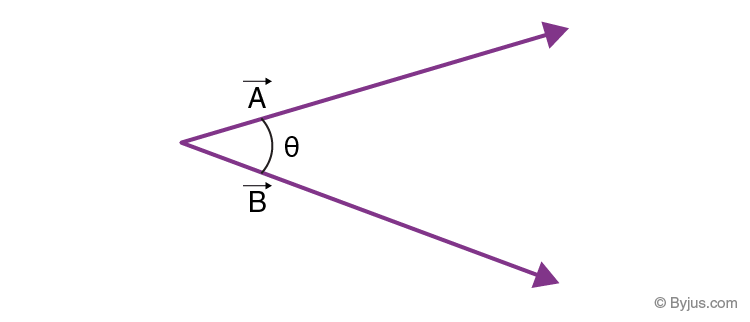# Angle Between Two Vectors

The vector quantities possess magnitude as well as direction, whereas scalar quantities have magnitude only, but not direction. A vector may be represented in the following form:

In two dimensions:

$$\begin{array}{l}\vec{A} = A_{x}i+A_{y}j\end{array}$$

Example: 2i+ 3j

In three dimensions:

$$\begin{array}{l}\vec{A} = A_{x}i+A_{y}j+A_{z}k\end{array}$$

Example: 2i+ 3j -7k

Two vectors may be inclined at an angle from each other as shown in the following figure:## Angle Between Two Vectors Formula

Before understanding the formula of the angle between two vectors, let us understand how to find a scalar product or dot product of two vectors.

Let us assume that two vectors are given such that:

$$\begin{array}{l}\vec{A} = A_{x}i+A_{y}j+A_{z}k\end{array}$$
and
$$\begin{array}{l}\vec{B} = B_{x}i+B_{y}j+B_{z}k\end{array}$$

Then dot product or scalar product of

$$\begin{array}{l}\vec{A}\end{array}$$
and
$$\begin{array}{l}\vec{B}\end{array}$$
can be calculated as:

$$\begin{array}{l}\vec{A}.\vec{B} = A_{x}B_{x}+ A_{y}B_{y}+A_{z}B_{z}\end{array}$$

The angle θ between the two vectors

$$\begin{array}{l}\vec{A}\end{array}$$
and
$$\begin{array}{l}\vec{B}\end{array}$$
can be calculated using the following formula:

$$\begin{array}{l}\theta=cos^{-1}\frac{\vec{A}.\vec{B}}{\left | \vec{A} \right |\left | \vec{B} \right |}\end{array}$$

Where

$$\begin{array}{l}\vec{A}.\vec{B}\end{array}$$
= Dot product of
$$\begin{array}{l}\vec{A}\end{array}$$
and
$$\begin{array}{l}\vec{B}\end{array}$$

$$\begin{array}{l}\left | \vec{A} \right |\end{array}$$
= Magnitude of vector A

$$\begin{array}{l}\left | \vec{A} \right |=\sqrt{A_x^2+A_y^2+A_z^2}\end{array}$$

$$\begin{array}{l}\left | \vec{B} \right |\end{array}$$
= Magnitude of vector B

$$\begin{array}{l}\left | \vec{B} \right |=\sqrt{B_x^2+B_y^2+B_z^2}\end{array}$$

## Cosine of Angle Between Two Vectors

The formula for finding cosine of angle between two vectors can be deduced by the formula of angle between two vectors

$$\begin{array}{l}\vec{A}\end{array}$$
and
$$\begin{array}{l}\vec{B}\end{array}$$
is
$$\begin{array}{l}\theta=cos^{-1}\frac{\vec{A}.\vec{B}}{\left | \vec{A} \right |\left | \vec{B} \right |}\end{array}$$

Therefore, the cosine angle between two vectors is given by

$$\begin{array}{l}\theta=cos^{-1}\frac{\vec{A}.\vec{B}}{\left | \vec{A} \right |\left | \vec{B} \right |}\end{array}$$

So, the cosine of the angle between two vectors can be calculated by dividing the dot product of the vectors by-product of their magnitudes.

Distance Between Two Vectors

Let us suppose that two vectors that are defined in two-dimensional space be:

$$\begin{array}{l}\vec{A} = A_{x}i+A_{y}j\end{array}$$
and
$$\begin{array}{l}\vec{B} = B_{x}i+B_{y}j\end{array}$$

Therefore, the distance between two vectors such as vector A and vector B is given as

$$\begin{array}{l}d =\sqrt{(A_{x}-B_{x})^{2}+ (A_{y}-B_{y})^{2}}\end{array}$$

Similarly, consider two vectors defined in three-dimensional space be:

$$\begin{array}{l}\vec{A} = A_{x}i+A_{y}j+A_{z}k\end{array}$$
and
$$\begin{array}{l}\vec{B} = B_{x}i+B_{y}j+B_{z}k\end{array}$$

Therefore, the distance between two vectors such as vector A and vector B is given as

$$\begin{array}{l}d =\sqrt{(A_{x}-B_{x})^{2}+ (A_{y}-B_{y})^{2}+ (A_{z}-B_{z})^{2}}\end{array}$$

## How to Find the Angle Between Two Vectors?

To find the angle between two vectors, one needs to follow the steps given below:

Step 1: Calculate the dot product of two given vectors by using the formula :

$$\begin{array}{l}\vec{A}.\vec{B} = A_{x}B_{x}+ A_{y}B_{y}+A_{z}B_{z}\end{array}$$

Step 2: Calculate the magnitude of both the vectors separately. Magnitude can be calculated by squaring all the components of vectors and adding them together and finding the square roots of the result.

Step 3: Substitute the values of dot product and magnitudes of both vectors in the following formula for finding the angle between two vectors, i.e.

$$\begin{array}{l}\theta=cos^{-1}\frac{\vec{A}.\vec{B}}{\left | \vec{A} \right |\left | \vec{B} \right |}\end{array}$$

## Angle Between Two vectors Example

Question: Calculate the angle between vectors 2i + 3j – k and i – 3j + 5k

Solution:

Let

$$\begin{array}{l}\vec{A}\end{array}$$
=2i + 3j – k and
$$\begin{array}{l}\vec{B}\end{array}$$
=i – 3j + 5k

Scalar product of

$$\begin{array}{l}\vec{A}\end{array}$$
and
$$\begin{array}{l}\vec{B}\end{array}$$
is

$$\begin{array}{l}\vec{A}. \vec{B}\end{array}$$
= 2 + (-9) + (-5) = – 12

Magnitude of

$$\begin{array}{l}\vec{A}\end{array}$$
is:

$$\begin{array}{l}\left | \vec{A} \right |=\sqrt{4+9+1}=\sqrt{14}\end{array}$$

Magnitude of

$$\begin{array}{l}\vec{B}\end{array}$$
is:

$$\begin{array}{l}\left | \vec{B} \right |=\sqrt{1+9+25}=\sqrt{35}\end{array}$$

The formula to find the angle between two vectors is

$$\begin{array}{l}\theta=cos^{-1}\frac{\vec{A}.\vec{B}}{\left | \vec{A} \right |\left | \vec{B} \right |}\end{array}$$

$$\begin{array}{l}\theta = cos^{-1}\frac{-12}{\sqrt{14}\sqrt{35}}\end{array}$$

$$\begin{array}{l}\theta = cos^{-1}\frac{-12}{3.64 \times 5.92}\end{array}$$

$$\begin{array}{l}\theta = cos^{-1}(-0.542)\end{array}$$

$$\begin{array}{l}\theta = 122. 82^{\circ}\end{array}$$

Therefore, the angle between two vectors 2i + 3j – k and i – 3j + 5k is

$$\begin{array}{l}122. 82^{\circ}\end{array}$$
.

For more Maths-related articles, register with BYJU’S – The Learning App and explore videos to learn with ease.